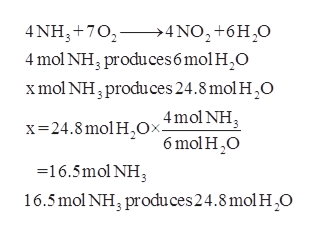# Given the equation: 4 NH3 + 7 O2 ----> 4 NO2 + 6 H2O(a) How many moles of NH3 must react if 24.8 moles of water are produced?(b) Determine the number of moles of H2O that would be formed from the combustion of86.0 grams of NH3.

Question
12 views

Given the equation: 4 NH3 + 7 O2 ----> 4 NO2 + 6 H2O

(a) How many moles of NH3 must react if 24.8 moles of water are produced?

(b) Determine the number of moles of H2O that would be formed from the combustion of
86.0 grams of NH3.

check_circle

Step 1

Moles of ammonia react if 24....help_outlineImage Transcriptionclose4 NH3 702>4NO2+6H20 4 mol NH, produces 6 mol H2O xmol NH, produces 24.8 mol H2O 4mol NH 6 mol H O x 24.8 mol HOx. -16.5mol NH 16.5mol NH3 produces24.8 mol H O fullscreen

### Want to see the full answer?

See Solution

#### Want to see this answer and more?

Solutions are written by subject experts who are available 24/7. Questions are typically answered within 1 hour.*

See Solution
*Response times may vary by subject and question.
Tagged in

### Physical Chemistry